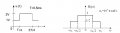# Linear time invariant system - find output signal if transfer function is known

#### xxxyyyba

Joined Aug 7, 2012
289
H!a) Calculate and sketch amplitude spectrum of u(t),
b) u(t) is input signal for linear time invariant system whose transfer function H(jw) is shown. Calculate output signal uo(t)

I completed task a), I got $$\small u(t)=\frac{3}{2}+\sum_{n=-\infty,n\neq 0}^{n=\infty}\frac{1}{2n\pi }(\sin{\frac{3n\pi}{2}}-\sin{\frac{n\pi}{2}})e^{jn\frac{2\pi}{0.5*10^{-3}}t},$$ where amplitude spectrum is $$\small F_n=|F_n|=\frac{1}{2n\pi }(\sin{\frac{3n\pi}{2}}-\sin{\frac{n\pi}{2}}).$$ Any suggestion about task b) ?

Last edited:

#### shteii01

Joined Feb 19, 2010
4,644
Use convolution.

Although u(t) is in time domain. H(jw) is in frequency domain. First you will have to choose which domain to use.

#### xxxyyyba

Joined Aug 7, 2012
289
Here is "step by step" tutorial from my book:
1. Define excitation in frequency domain: Xn or X(jw)
I did it already, $$\small u(t)=\frac{3}{2}+\sum_{n=-\infty,n\neq 0}^{n=\infty}\frac{1}{2n\pi }(\sin{\frac{3n\pi}{2}}-\sin{\frac{n\pi}{2}})e^{jn\frac{2\pi}{0.5*10^{-3}}t},$$ where amplitude spectrum is $$\small F_n=|F_n|=\frac{1}{2n\pi }(\sin{\frac{3n\pi}{2}}-\sin{\frac{n\pi}{2}}).$$ (Xn from book is Fn here).
2. Multiply transfer function of system and spectrum of excitation (H(jw)Xn or H(jw)X(jw) to get response in frequency domain: Yn or Y(jw)
But my transfer function is piecewise defined, it's 1 in interval w<wc/2 and 1/2 between wc/2 and wc.
3. Apply inverse Fourier transform to find analytical expression for output signal

Last edited:

#### t_n_k

Joined Mar 6, 2009
5,455
I would think there is another simple approach - at least to the extent that one may gain some intuitive understanding of what happens.
As the OP has indicated, given the function u(t) is periodic one can derive the Fourier series for the function. The amplitudes at the progressive (odd) harmonics are well defined ad infinitum.
The LTI system to which u(t) is input, has sharp amplitude / gain scaling transitions at 0.5*Wc [5*Pi Mrads/sec] (gain goes to 0.5) and at Wc [10*Pi Mrads/sec] (gain goes to zero). The frequency terms in the Fourier series for u(t) which lie between W=0 and 0.5*Wc will suffer no amplitude change - including the DC offset. The frequency terms in the Fourier series which lie between 0.5Wc and Wc will be attenuated by a factor of 0.5. The frequency terms in the Fourier series which lie between Wc and infinity will be cut off.
I presume one could therefore express the output in terms of the three frequency regions of interest - say in terms of the particular range of harmonics which are coincident with or near the known transition points (frequencies) between the regions. I dont see how one could find an inverse form (frequency domain to time domain) for the modified input to give a closed time domain form.

•xxxyyyba

#### MrAl

Joined Jun 17, 2014
8,353
Hi,

I dont see anything that states specifically if the input pulse u(t) is periodic or just one pulse. That would be the first thing we'd have to know. Usually periodic signals are drawn with at least two or three cycles shown but they might have wanted to save space.
Are there other examples in the same book, or do they state it one way or the other?

•xxxyyyba

#### xxxyyyba

Joined Aug 7, 2012
289
It's periodic signal, they just didn't draw it correctlyThere are no other examples of this type in my book. Usually they ask as to calculate output y(t) for input x(t) (represented in terms of Fourier series) and some transfer function of jw (H(jw)) but H(jw) wasn't defined on this way so far.

#### MrAl

Joined Jun 17, 2014
8,353
Hello again,

Do they usually ask for the continuous Fourier Transform or do they let you use a series?
Also, do they give any results?
I ask because i am wondering how they want you to approach this problem.

#### xxxyyyba

Joined Aug 7, 2012
289
We used only series... I will contact my teacher to reveal me trick. I will write result here.

#### MrAl

Joined Jun 17, 2014
8,353
Hello again,

Ok that sounds good. It seemed better to use the continuous Fourier Transform but then we'd get a result like:
pi*d(w)+1/jw
for the pulse and that would have to be applied twice (d(w) is the impulse function), or something like:
A*T*(sin(wt/2))/(w*T/2))*e^(-j*2*pi*f*t0)

both of which are totally different than the series, and then the integration might go over 0 to wc treating the filter as an ideal lowpass and ideal bandpass over different frequency ranges.

I also had to wonder about applying an integer frequency range and it would seem better to start you off with an ideal lowpass rather than two ideal lowpass's or a lowpass and a bandpass.

I look forward to seeing more information here...but in the mean time here is a quick shot of that pulse put through a low pass filter but where T=2*pi.

Last edited:

#### MrAl

Joined Jun 17, 2014
8,353
Hi again,

While we were waiting i found the response for a single pulse through an ideal low pass filter, which is also interesting.

The time response in two parts is:
v1(t)=Si(2*pi*B*t)/pi
v2(t)=Si(2*pi*B*(t-T))/pi

and the entire response is then:
v(t)=v1(t)-v2(t)

Here, Si(x) is the "sine integral" function, and B is the bandwidth of the ideal filter, and t is time, and T is the length of the pulse, and the pulse starts at t=0 so it ends at t=T.

The response to the original pulse (if it was a single pulse) and original filter of this thread will be slightly different because the pulse is time shifted and offset, and the filter is the combination of an ideal low pass filter and ideal band bass filter (or possibly two low pass filters).

A partial graph of the response with B=1 and T=1/2 is shown in the attachment. Note how very different it appears compared to the periodic pulse response.

One thing we might note right away is that the response is not causal so it can never be filtered this way in real time.

Last edited: